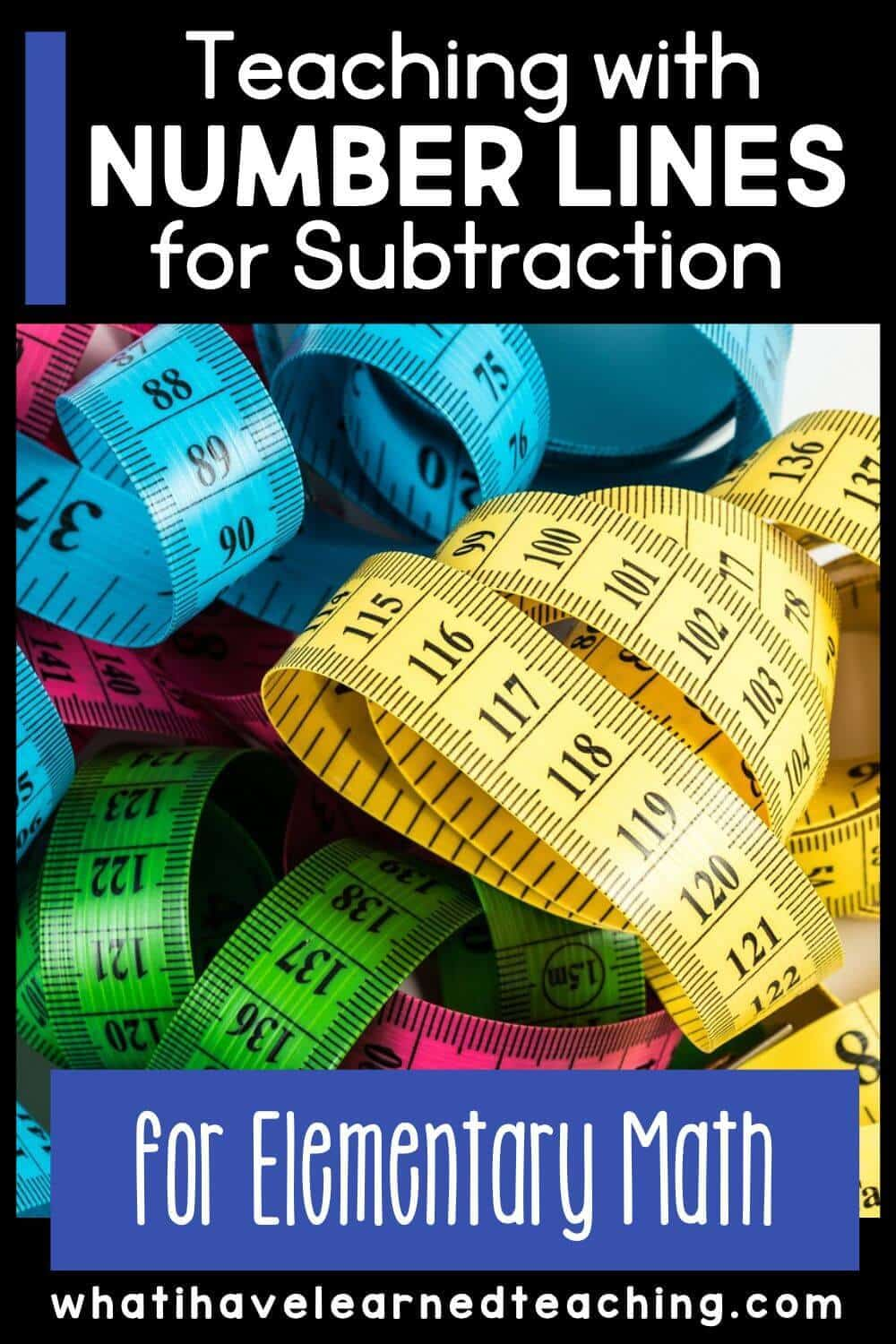Tuesday June 6, 2023
Latest:
179 total views

A number line is a powerful tool that can be used to teach a variety of mathematical concepts. They are a great visual tool that can be used to help students understand and learn single-digit, two-digit, and three-digit addition and subtraction. How can use use number lines for subtraction?

In this blog post, we’ll focus on how number lines can be used to teach subtraction in second grade. We’ll provide an overview of how to teach students to subtract using a number line, as well as some strategies and tips for using number lines in the classroom.

## Teaching Students To Subtract On A Number Line

There are a few different ways that teachers can introduce subtracting numbers on a number line to their students.

### Review Addition on a Number Line

One way is to start with a review of addition on a number line. This will help students understand the concept of movement along a number line, as well as the concept of “taking away” or subtracting. Relating addition to subtract will anchor the new concept on known information. When you move from single digit to two-digit, start with addition. Model it on a number line, then move to subtraction.

### Subtract by Finding the Distance Between Two Numbers

It can be helpful for students to see that the answer to a subtraction problem is always the distance between the two numbers on the number line. Find two numbers on the number line and then have students count the distance between them. Do this repeatedly.

### Subtract by Counting Backward on a Number LIne

Another way to introduce subtraction on a number line is to have students begin with a whole number and then count backward from that number by the desired amount. For example, if you want students to find the answer to the subtraction problem 9 – 3, you could have them begin with the number 9 on the number line and then count back 3 units. This would take them to the number 6, which is also the answer to the problem 9 – 3.

## Strategies And Tips For Using Number Lines for Subtraction in the Clasroom

Number lines can be used in a variety of ways in the classroom beyond just teaching addition and subtraction problems. Here are a few number line strategies:

• Use a number line as part of your daily calendar routine. Have students count forwards or backward by ones, twos, or fives each day as you move along the number line together.
• Incorporate movement into your math lessons by having students walk along or jump along a human number line when finding answers to addition or subtraction problems.
• Use open-ended tasks and prompts that allow for student choice and creativity when working with number lines. For example, you might ask students to create their own games or puzzles involving addition and subtraction on a number line.
• Hang a blank number line in your classroom and invite students to add new elements to it (e.g., markings for important holidays or events) throughout the year as they learn more about measurement and place value concepts.
• Give students opportunities to work with small groups or partners when using number lines to encourage collaborative learning and student engagement.

## Daily Math Number of the Day Activity

One of the key ways I have taught my students to subtract using a number line is to do it repeatedly. We worked it into our daily math routine. At the beginning the year we chose easier numbers and modeled number lines with one-digit numbers. As we learned more math, we increased the complexity of the numbers on the number line.

## Ways to Use Number Lines for Addition and Subtraction

Teachers can use variety of number line strategies to help their students work through complex mathematical problems. This blog post goes into ways to use a number line for addition and subtraction of whole numbers.

## Number Line Strategy for Three-Digit Subtraction

Are you teaching three-digit subtraction with regrouping? Or having to cross hundreds and tens? A number line is a great tool to use for three-digit subtraction. This blog post shows you a unique teaching strategy for using number lines with three-digit numbers.

## Vertical Number Lines

Have you ever thought of using a vertical number line? Sometimes just turning the number line on its side will help students see it a different way. This number line strategy mimics a thermometer.

Find out how I use a vertical number line in my classroom in this blog post.

Number lines are versatile tools that can be used in many different ways in the classroom. We hope this blog post has given you some ideas for how you can use number lines in your own second-grade classroom when teaching addition and subtraction problems! Thank you for reading!### News

Some days, you simply have to put the books aside and head outside. These nature crafts are the perfect…